# Lindelöf summation method

(diff) ← Older revision | Latest revision (diff) | Newer revision → (diff)
Jump to: navigation, search

A semi-continuous method for summing series of numbers and functions (cf. Summation methods), defined by the system of functions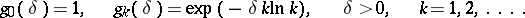The series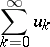is summable by the Lindelöf summation method to the sumif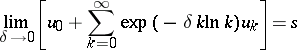and the series under the limit sign converges. The method was introduced by E. Lindelöf  for the summation of power series.

The Lindelöf summation method is regular (see Regular summation methods) and is used as a tool for the analytic continuation of functions. Ifis the principal branch of an analytic function, regular at the origin and representable by a series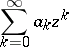for small, then this series is summable by the Lindelöf summation method toin the whole star of the function(cf. Star of a function element), and it is uniformly summable in every closed bounded domain contained in the interior of the star.

Of the summation methods determined by a transformation of a sequence into a sequence by semi-continuous matrices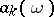of typewhere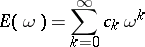is an entire function, Lindelöf considered the case whenA matrix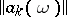constructed from an entire function of this kind is called a Lindelöf matrix.